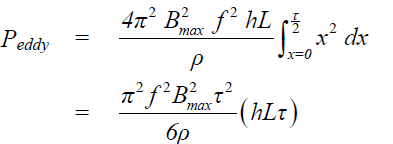# Eddy Current Loss Formula

### What is eddy current?

When the conductor is placed in the varying magnetic field the localized current starts flowing in the conductor is called the eddy current. The energy loss wasted in the form of heat energy in the conductor caused by flow of eddy current is known as eddy current loss.

The eddy current loss can be minimized by use of thin laminated silicon steel sheets in place of solid steel sheet. The various factor like magnetic flux density, frequency, electrical properties of the material and thickness of the laminated sheets affect the eddy current loss. The derivation of the eddy current loss formula gives in-depth overview of the factors on which eddy current loss depends.The formula of the eddy current loss(P) is as given below.

Let us derive the formula of the eddy current loss for better understanding of the eddy current loss in a steel core.

## Derivation of eddy current loss formula

With an use of thin laminated sheets, the area of the eddy current path reduces and thus the resistance of the path gets increased. With an increased resistance the potential developed at two points of the lamination gets lowered and as a result the magnitude of eddy current gets reduced and eddy current loss gets reduced.
Let the length, height and thickness of the laminated sheet is L,h and𝞃 respectively.The thickness of the sheet 𝞃 is very very less than the length(L) and height(h) of the sheet.Let the sinusoidal time varying magnetic field is there perpendicular to the area formed by 𝞃 and h.
The varying magnetic field perpendicular to area formed by 𝞃 and h is;
B= BmaxSinωt

Let us consider a small elemental closed loop path PQRSP of thickness dx and distance of the small element from origin is x. The voltage will be induced in this close loop similar to as induce in a single turn coil.

Area of the loop PQRS(A)  = 2hx   ——-(1)

Theflux density B = Φ/A

The flux crossing the loop PQRSP =

Φ= BA

ΦBmaxSinωt2hx        ——————-(2)
RMS voltage induced in the loop

E=  4.44 Φmax

E= f Φmax            [2Π=4.44]

E=2 Π f Bmax A

E=  2Π f Bmax2 hx   ——————-(3)

Resistance of the eddy current path

R = ρ * ( 2h+4x)/ L dx     ————–(4)

The eddy current loss(dP) in a small elemental loop dx

dP = E2/R

dP = E2 Ldx/[ρ * ( 2h+4x)]     ——–(5)

Since thickness of the sheet  is very less compared to height of the sheet and x dimension can be ignored.

dP = E2 Ldx/(ρ*2h)

dP = [√2ΠfBmax2hx]2 Ldx/(ρ*2h)]

dP = [4ΠfBmax2hx]2 Ldx/(ρ*2h)]

dP = 2Bmax2f2hL/ρ       ———-(6)

Total eddy current loss =The volume of sheet (V) =hL𝞃

Eddy current loss per unit volume =

Peddy = Π2Bmax22𝞃2/ ——–(7)
Peddy= KfBmax2𝞃2   —————(8)
Where,
Ke = Π2/6ρ
Ke is called the eddy current coefficient.
Thus the eddy current loss per unit volume is ;
• Proportional to the square of the frequency
• Proportional to the square of the flux density
• Proportional to the square of the thickness of the laminated sheet
• Inversely proportional to the resistivity of the material

## How to minimize eddy current loss?

The eddy current loss depends on the thickness of steel core. The thin laminated sheet can be used in place of one solid piece of a steel core.The eddy current loss in the steel core gets minimized if the thin laminated steel sheets which are electrically insulated are used. The thin laminated sheet has higher resistance. Therefore, less voltage is induced in the sheet when sheet is placed under varying magnetic field. The low induced voltage with thin sheet lead to lower eddy current and as a result, the heat loss(I2R) reduce in the thin laminated sheet.

The other electrical quantity that affects the eddy current loss is the ratio of the voltage and frequency.The eddy current loss remains constant if the following electrical quantities remains constant.
• Voltage
• Frequency
If the voltage and frequency is constant the flux density will also remains constant because the flux density is proportional to the ratio of V/f.

### Applications of Eddy Current

The eddy current is undesired in the motor, transformer as it cause heat loss which is wastage of energy. However,  the eddy current can be usefully used in the following applications.

• Damping:  The coil of the measuring instrument is mounted in the light metal frame. When the coil and the pointer rotate in the permanent magnetic field, the eddy current induces in the metal frame which produce the damping torque.
• Electric Brakes: When a stationary magnetic field is applied to a rotating drum, the eddy current set up in the drum produce the braking torque. The brakes are known as eddy current brakes.
• Induction Furnace: In induction furnace, the metal which is to be heated up is placed in the rapidly changing magnetic field produced by high frequency current. The eddy current is set up in the metal piece and thus the metal get heated up. The induction bearing heater also functions on the same principle.
• The effect of eddy current is used for providing braking torque in induction type energy meters.
• Eddy current instruments are used for detecting cracks in the metal parts.

Related Posts

1.